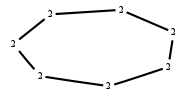# The Markov Bases Database

## C7_bin

The binary graphical model/graph model of C7.

It is a hierarchical model of 7 variables. The dimension of the model is 14.The cardinality of the statespace is 128.

### Properties of the Markov basis

 Markov degree 4 3472

 degree # of gen. 2 4 2912 560

The model has the following properties:

• All variables are binary.
• It is a graph model and a graphical model.
• The semigroup is normal.

The following properties of the model are unknown:

• The minimal Markov basis may be unique.### FILES

 Markov basis: sufficient statistics matrix: C7_bin.mar (1.27 Mb) C7_bin.mat (7.03 kb) C7_bin.mod (62 b) C7_bin.tar.gz (26.16 kb)

Wrong or missing information? Write us an email.

page last update on November 15 2020. ->Contact